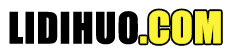# Python NumPy教程NumPy是Python中科学计算的基础包。它是一个Python库，提供多维数组对象，各种派生对象（如掩码数组和矩阵），以及用于数组快速操作的各种API，有包括数学、逻辑、形状操作、排序、选择、输入输出、离散傅立叶变换、基本线性代数，基本统计运算和随机模拟等等。

NumPy 的前身 Numeric 最早是由 Jim Hugunin 与其它协作者共同开发，2005 年，Travis Oliphant 在 Numeric 中结合了另一个同性质的程序库 Numarray 的特色，并加入了其它扩展而开发了 NumPy。NumPy 为开放源代码并且由许多协作者共同维护开发。
NumPy包的核心是 ndarray 对象。它封装了python原生的同数据类型的 n 维数组，为了保证其性能优良，其中有许多操作都是代码在本地进行编译后执行的。
NumPy数组 和 原生Python Array（数组）之间有几个重要的区别：
NumPy 数组在创建时具有固定的大小，与Python的原生数组对象不同,更改ndarray的大小将创建一个新数组并删除原来的数组。 NumPy 数组元素需具有相同的数据类型，因此在内存中的大小相同。 NumPy 数组有助于对大量数据进行高级数学和其他类型的操作。通常，这些操作的执行效率更高，比使用Python原生数组的代码更少。 越来越多的基于Python的科学和数学软件包使用NumPy数组，但它们在处理之前会会将输入的数组转换为NumPy的数组。

## NumPy 应用

NumPy 通常与 SciPy一起使用，这种组合广泛用于替代 MatLab，有助于我们通过 Python 学习数据科学或者机器学习。 SciPy 是一个开源的 Python 算法库和数学工具包。 SciPy 包含的模块有最优化、线性代数、积分、插值、特殊函数、快速傅里叶变换、信号处理和图像处理、常微分方程求解和其他科学与工程中常用的计算。 Matplotlib 是 Python 编程语言及其数值数学扩展包 NumPy 的可视化操作界面。它为利用通用的图形用户界面工具包。

## 相关资料

NumPy 官网：http://www.numpy.org NumPy 源代码：https://github.com/numpy/numpy SciPy 官网：: https://www.scipy.org SciPy 源代码：: https://github.com/scipy/scipy Matplotlib 源代码：: https://matplotlib.org Matplotlib 源代码：: https://github.com/matplotlib/matplotlib

## Numpy的简单实例

# 1、安装包
\$ pip install numpy
# 2、进入python的交互式界面
\$ python -i
# 3、使用Numpy
>>> from numpy import *
>>> eye(4)
# 4、输出结果
array([[1., 0., 0., 0.],
[0., 1., 0., 0.],
[0., 0., 1., 0.],
[0., 0., 0., 1.]])